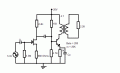# Solve for Vo. Multi stage amplifier circuit Common source JFET, Common emitter BGT.

#### electrogirl

Joined May 2, 2010
47
Calculate the voltage output for the amplifier shown.How should I solve for the output voltage?

My strategy is to calculate the voltage gain of each stage then solve for the overall voltage gain. Is this right?

Avtotal = Av1 X Av2
then,
Vo = Avtotal X Vi

Edited: Idss = 10mA, Vp = -4V

Last edited:

#### bertus

Joined Apr 5, 2008
21,603
Hello,

Please post the schematic as an .PNG or PDF file.

Not every one (like me) can read the .sch file.

Bertus

•electrogirl

#### retched

Joined Dec 5, 2009
5,208
Sorry electrogirl, I couldn't open it either.

•electrogirl

#### PRS

Joined Aug 24, 2008
989
Hi, electrogirl! Perhaps you can save it as a JPEG file using Paint?

•electrogirl

#### electrogirl

Joined May 2, 2010
47
Hello,

Please post the schematic as an .PNG or PDF file.

Not every one (like me) can read the .sch file.

Bertus
it's now in gif format, perhaps you can check it now. ^_^

#### PRS

Joined Aug 24, 2008
989
it's now in gif format, perhaps you can check it now. ^_^
Without parameters for the JFET I see no way to calculate its gain. Are you sure they were not given? Was a specific JFET such as an MPF102 given so we can look up the manufacturer's data sheet? Or is this a lab project where you can experimentally determine the parameters?

•electrogirl

#### electrogirl

Joined May 2, 2010
47
Without parameters for the JFET I see no way to calculate its gain. Are you sure they were not given? Was a specific JFET such as an MPF102 given so we can look up the manufacturer's data sheet? Or is this a lab project where you can experimentally determine the parameters?
I'm sorry there was an error on the problem that I've posted, I wasn't able to copy the given correctly Idss = 10mA Vp = -4V

#### Jony130

Joined Feb 17, 2009
5,244
hmm, voltage gain of the firs stage is equal:

Au1 = ( 2.4K||15K||4.7K||(201*re2) ) / (1/gm + 680)

re2 = 26mV/Icso, to find gm we need to know Vgs
So we need to solve this quadratic equation:

$$Idss * ( 1 - \frac{Vgs}{Vp})^2 = - \frac{Vgs}{Rs}$$
Rs = 680Ω
So Vgs = -1.89V; Id=2.78mA

The gain of a second stage is equal:

Au2 = (Rc||ro)/re2

So you need to find Ic and Rc, and this can help you find RcSo you have enough information to solve this problem.

•electrogirl

#### PRS

Joined Aug 24, 2008
989
Here's my solution for the gain of the first amp. I didn't show all my work, but there's enough for you to see the method.

The gain of the second amp will be the resistance seen by the collector divided by the resistance at the emitter. And then 4:1 step down to the load. Be sure to reflect the resistance of the load into the primary of the transformer when you calculate the gain at the collecter.

#### Attachments

•electrogirl

#### electrogirl

Joined May 2, 2010
47
Here's my solution for the gain of the first amp. I didn't show all my work, but there's enough for you to see the method.

The gain of the second amp will be the resistance seen by the collector divided by the resistance at the emitter. And then 4:1 step down to the load. Be sure to reflect the resistance of the load into the primary of the transformer when you calculate the gain at the collecter.
Thank you so much for resolving this problem!

Last edited:

#### PRS

Joined Aug 24, 2008
989
Thank you so much for resolving this problem!
Actually, that was the solution for the first stage only. But you're most welcome. The second stage is kind of complicated so I solved that, too. If you were also given the inductance of the primary of the transformer or its impedance, then that has to be included. Without it I assumed it was large enough to ignore.

The main thing is to study the solution and learn from it. Also, carefully check my work; it may have mathematical errors. I'm quite absent-minded these days.

Good luck!#### Attachments

•electrogirl